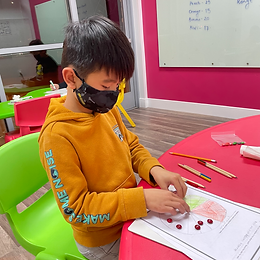Ms. Caitlyn

Target 1​

Lesson Type:

New

Data Probability

:

Interpreting Data

Construct and read graphs from given information to solve problems.

1:

Recognize that circle graphs are used to compare the parts of a whole.

2:

Construct and read pie (circle) graphs.

3:

Define circle graph.

4:

Recognize that a circle graph provides a visual representation of the whole and its parts.

5:

Determine the fraction that each part of the circle graph represents.

5th

Vocabulary:

Circle Graph: Also known as a pie chart, a circle graph provides a visual representation of a whole and its parts.
Key/Legend: Explains and defines symbols and colors used in a graph or chart.
Fraction: A part of a whole.

Activities:

• Students went on a scavenger hunt to find various fruit.
• Students organized the fruit that they found into a circle graph.
• Students made a key for their circle graph and wrote the fraction of each fruit beside it.Home Exploration

Guiding Questions:Absent Students:

Zachary

Target 2

:

1:

Determine the outcomes and probabilities for experiments.

2:

Understand that the likelihood of an event occurring can be described numerically and used to make predictions.

3:

Identify the possible outcomes of an event.

5th

Vocabulary:

Probability: The likelihood of an event to occur.

Activities:

• Students explored probability with M&Ms.Home Exploration

Guiding Questions:Target 3

:

Vocabulary:

Activities:Home Exploration